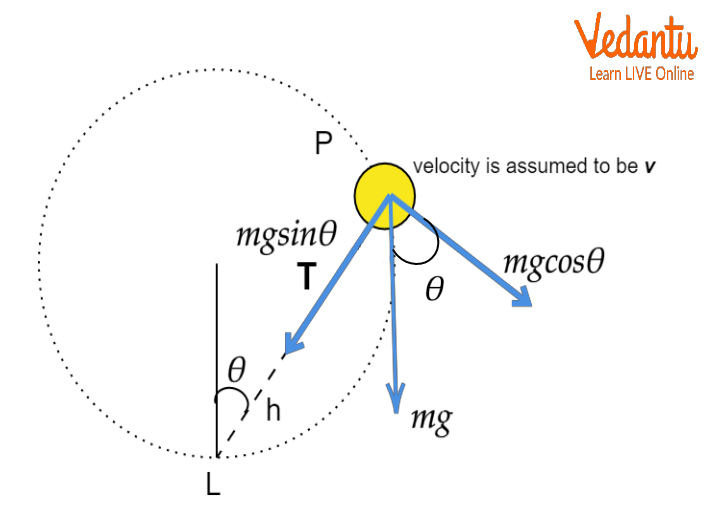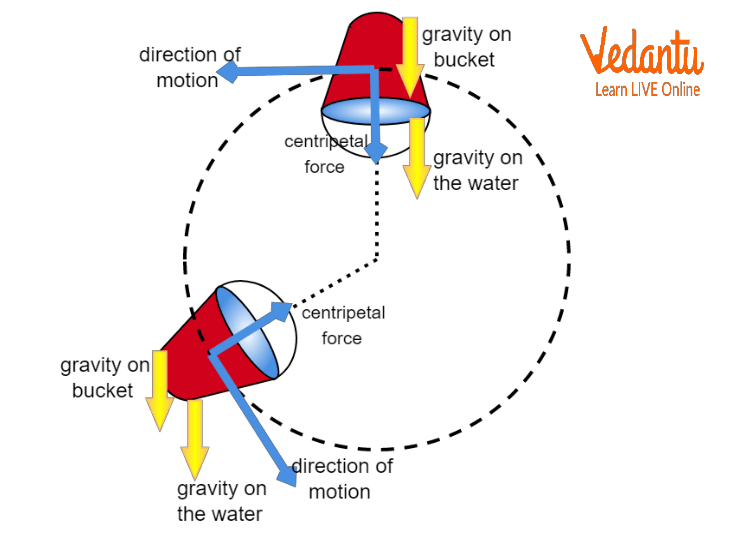Courses
Courses for Kids
Free study material
Free LIVE classes
More

# Motion in a Vertical Circle - JEE Important TopicLIVE
Join Vedantu’s FREE Mastercalss

## Vertical Circular Motion

The effect of gravity is considered when the motion of an object in a vertical circle is studied. The gravitational field of the earth influences the velocity of the body and the tension in the string, due to which the magnitude of velocity and tension keeps changing continuously. The motion in a vertical circle is considered non-uniform as the influence is observed the most at the lowest and the least at the highest point of the path.

## Examples of Motion in a Vertical Circle

Some of the examples of vertical circular motion are listed below.

1. A roller coaster

3. A bucket of water on string

## Derivation of Velocity of Particle at Any Point

Suppose a body of mass ‘m’ is under motion in a vertical circle, and is tied to one end of a string. We know that the acceleration of the body will increase as it goes towards the lowest point on the vertical circle and it will decrease as it goes towards the highest point on the circle. Therefore, the speed of the body varies continuously, being maximum at the lowest and minimum at the highest point. The body undergoes non-uniform motion. Here, the weight of the body, (mg) will always act downwards and is not influenced by the position of the body in the path.

At any point P, the velocity of the body is assumed to be ‘v’. The point L represents the bottommost point on the vertical circle, and ‘h’ represents the vertical distance between the point P and L.Velocity of Particle in Vertical Motion

Then, by Energy Conservation Law, we have

$m{{v}^{2}}+2mgh=m{{u}^{2}}$

${{v}^{2}}={{u}^{2}}-2gh$

$v=\sqrt{{{u}^{2}}-2gh}\text{ }$   ……. 1

The above equation (1) gives the velocity of anybody undergoing motion in a vertical circle.

## Derivation of Tension in the String

At the point P in the above case, a centripetal force is acting. Then, we have

$T-mg\cos \theta =\dfrac{m{{v}^{2}}}{r}$                         ……. 2

$T=mg\cos \theta +\dfrac{m{{v}^{2}}}{r}$

From the diagram, we have

$\cos \theta =\dfrac{r-h}{r}$

Using the above value in equation (2) gives

$T=mg\left( \cos \theta =\dfrac{r-h}{r} \right)+\dfrac{m{{v}^{2}}}{r}$

$T=\dfrac{m}{r}\left[ g\left( r-h \right)+{{v}^{2}} \right]$

Since, we know that

${{v}^{2}}={{u}^{2}}-2gh$

Therefore, we have

$T=\dfrac{m}{r}\left[ gr-gh+{{u}^{2}}-2gh \right]$

$T=\dfrac{m}{r}\left[ {{u}^{2}}-3gh+gr \right]$       ……. 3

The above equation (3) gives the tension of the string.

Establish a relation between tension in the string at the highest and that at the lowest point of the circle.

Let’s discuss each of the cases as shown below

1. When the body reaches the lowest point of the vertical circle

We know that $T=\dfrac{m}{r}\left( {{u}^{2}}-3gh+gr \right)$.

At the lowest point, we will have $h=0$.

Therefore, we have

${{T}_{L}}=\dfrac{m}{r}\left( {{u}^{2}}+gr \right)$

1. When the body reaches the highest point of the vertical circle

At the highest point, we will have $h=2r$

Therefore, we have

${{T}_{H}}=\dfrac{m}{r}\left( {{u}^{2}}-3g\left( 2r \right)+gr \right)$

${{T}_{H}}=\dfrac{m}{r}\left( {{u}^{2}}-5gr \right)$

Using the above two equations, we will get

${{T}_{L}}-{{T}_{H}}=\dfrac{m}{r}\left( {{u}^{2}}+gr \right)-\dfrac{m}{r}\left( {{u}^{2}}-5gr \right)$

${{T}_{L}}-{{T}_{H}}=6mg$

## Practical Applications of Motion in a Vertical Circle

1. Let us consider a bucket filled with water. Suppose it is rotated about a vertical circle in a manner that its velocity at the bottommost point will be equal to or greater than $\sqrt{5gr}$. In this situation, the water inside the bucket will not be spilled out even if the bucket reaches the topmost point of the circle.

2. Due to a similar situation, any individual who is not tied to the seat in an airplane, does not fall down when moving along the vertical circle.

3. It is observed that in a circus, the motorcyclists are able to perform the stunts with the motorcycle undergoing the motion in a vertical circle.

Let’s discuss one of the applications in detail.

## Whirling of a Water Filled Bucket

Two forces act on the bucket, i.e., the gravitational force on the bucket and the tension acts due to the string. Both these forces act in the downward direction at the point when the bucket reaches the highest point of the vertical circle. Now, let’s consider the water inside the bucket, here the gravitational force acts in the downward direction, and a normal force directed downwards from the bucket replaces the tension. Both cases can be analyzed similarly, so we will consider the water inside the bucket.

Now, we assume the direction of the acceleration to be the positive direction, that is directed towards the center of the vertical circle. At the topmost point of this vertical circular path, both the positive direction and acceleration will be directed in the downwards direction. There is no need to divide the acting forces in their components. Now, we apply the third law of Newton.

$F=ma$Whirling of Water Filled Bucket

We can determine the left-hand side, and the right-hand side can be written in the

general form of circular-motion. Thus, we have

$+mg+{{F}_{N}}=+\dfrac{m{{v}^{2}}}{r}$

Solving the above equation further, we get

${{F}_{N}}=\dfrac{m{{v}^{2}}}{r}-mg$

If the first expression on the right-hand side is greater than the second expression, i.e. if the normal force (FN) is non-negative, then there are no chances of the water being spilled on us. The water can lose contact with the bucket and starts to spill out only if the normal force becomes equal to zero. When the normal force becomes equal to zero, then the safe speed of the bucket at the highest point of the vertical circle becomes minimum.

$0=\dfrac{mv_{\min }^{2}}{r}-mg$

The above equation gives

${{v}_{\min }}=\sqrt{gr}$

## Conclusion

• The motion in a vertical circle is considered nonuniform as the influence is observed the most at the lowest and the least at the highest point of the path.

• Some of the examples are roller coasters, cars on hilly roads, etc.

• The velocity of anybody undergoing motion in a vertical circle is given by $v=\sqrt{{{u}^{2}}-2gh}$.

• The tension of the string is given by $T=\dfrac{m}{r}\left( {{u}^{2}}-3gh+gr \right)$

Last updated date: 30th Sep 2023
Total views: 3.3k
Views today: 0.03k

## FAQs on Motion in a Vertical Circle - JEE Important Topic

1. List some of the applications of motion in a vertical circle.

Some of the practical applications of motion in a vertical circle are listed below.

1. Let us consider a bucket filled with water. Suppose it is rotated about a vertical circle in a manner that its velocity at the bottommost point will be equal to or greater than $\sqrt{5gr}$. In this situation, the water inside the bucket will not be spilled out even if the bucket reaches the topmost point of the circle.

2. Due to a similar situation, any individual who is not tied to the seat in an airplane, does not fall down when moving along the vertical circle.

3. It is observed that in a circus, the motorcyclists are able to perform the stunts with the motorcycle undergoing the motion in a vertical circle.

2. What will be the minimum velocity of the body when it is at the highest lowest point on the vertical circle.

We discuss both the cases as shown below.

(a) This is the least velocity of the body that the body will require to move around the vertical circular path once. This means that the tension at the highest point must be greater than or equal to zero.

${{T}_{H}}\ge 0$

$\dfrac{m}{r}\left( {{u}^{2}}-5gr \right)\ge 0$

${{u}^{2}}\ge 5gr$

$u\ge \sqrt{5gr}$

(b) When the body is at the highest point in the circle, then $h=2r$.

We know that

$v=\sqrt{{{u}^{2}}-2gh}$

$v=\sqrt{\sqrt{5gr}-2g\left( 2r \right)}$

$v=\sqrt{5gr-4gr}$

$v=\sqrt{gr}$

The above two equations give the minimum velocities of the body at the lowest and highest points of the circle.